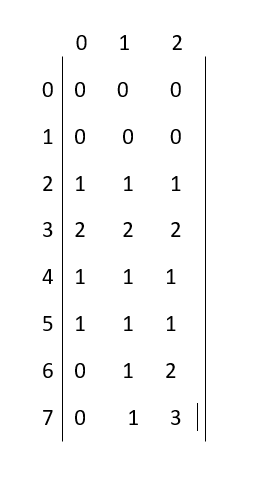×

Search anything:

# Number of ordered pairs such that (A[i] & A[j])=0

#### Algorithms Dynamic Programming (DP)Get this book -> Problems on Array: For Interviews and Competitive Programming

Reading time: 30 minutes | Coding time: 10 minutes

Given an array A[] of n integers, find out the number of ordered pairs such that (A[i]&A[j])=0,where 0<=(i,j)<n.Here,we are considering (i,j) and (j,i) to be different.There are some constraints in this problem: 1<=n<=10^4 and 1<=A[i]<=10^4. We can either go about it by brute-forcing through all possible pairs which will require a lot of time and calculations i.e. about O(n^2) or we can make use of dynamic programming to break this down into smaller sub-problems and use the result of each to build up to our solution.

To understand the dynamic programming approach let's just first learn some of the basic concepts.

## Bitwise AND set of a number n

Bitwise AND set of a number n is all posssible numbers i smaller than or equal to n such that (n&i) is equal to i.So,here we can say i will be a bitwise subset of mask n if (n&i)==i.

Example:

``````Input : n=9
Output : 0,1,8,9
Explanation : 0&9=0
1&9=1
2&9=0
3&9=1
4&9=0
5&9=1
6&9=0
7&9=1
8&9=8
9&9=9
``````

## Method

In the dynamic programming approach, we use a hash table(hash[])to count the occurrence of every element.Here,key observations are the constraints,the maximum that an array element can be is 10^4,so calculating the mask upto 2^15 will be enough.We will take a 2d-array dp[][], where the row represents the mask and the column represents the bit-no(bit-no of last bit will be considered as 0).We will iterate over 15 bits(let it be N) only because maximum mask value posssible is 2^15.dp[mask][i] will store the number of subsets of mask which differ from mask in first i bits.

For calculating number of subsets of a particular mask,

Let's consider the i-th bit to be 0,then no subset can differ from the mask in the i-th bit as it would mean that the numbers will have a 1 at the i-th bit where the mask has a 0 which would mean that it is not a subset of the mask.So,we can conclude that the numbers now differ in the first (i-1) bits only.Hence,

``````dp[mask][i] = dp[mask][i-1]
``````

Let's consider the i-th bit to be 1,then it can be divided into two non-intersecting sets.One set containing numbers with i-th bit as 1 and differing the mask in the next (i-1) bits,another set containing numbers with i-th bit as 0 differing from mask(xor)(2^i) in next (i-1) bits.Hence,

``````dp[mask][i] = dp[mask][i-1] + dp[mask (xor) (2^i)][i-1]
``````

• If the last bit is 0:
``````dp[mask] = hash[mask]
``````
• If the last bit is 1:
``````dp[mask] = hash[mask] + hash[mask (xor) 1]
``````

Let's see how we can fill the dp array with the help of psuedocode:

`````` Let N=15
create a hash table and store the occurrences of the element.
create a dp array, dp[1<<N][N+1]
initialize dp with 0.
else
for(i=1 to N+1){
else
}
}
``````

## ALgorithm

1. Create a 2d-array dp[][] and store the number of subsets of mask(present in the given array)which differ from mask in first i bits for each mask(as explained above).

2. Create a variable ans and for every array element add the number of subsets dp[temp] to ans,where temp is the complement of the given array element.
here,temp=((1<<15)-1)(xor)A[i].

3. Return ans.

As we know complement of a number gives 0 on applying bitwise & operator.So,obviously subset of the complement will also return 0.

Let's take an example:

Suppose A[i]=5 in binary it is 101.Suppose N=3 in this case,now reverse of 101 is 010 which on applying bitwise & gives 0.So,(1<<3) gives 1000 which on subtraction from 1 gives 111.111(xor)101 gives 010 which is the reversed bit.So,we can conclude that dp[temp] will have the number of subsets that returns 0 on applying bitwise & operator.

## Implementation

`````` #include<bits/stdc++.h>
using namespace std;

const int N=15;

//Function to count pairs
long long count(int A[],int n){
unordered_map<int,int> hash;
long long dp[1<<N][N];

//Initialize dp to 0
for(int i=0;i<(1<<N);i++){
for(int j=0;j<N;j++){
dp[i][j]=0;
}
}

//Count the frequency of every element
for(int i=0;i<n;i++){
hash[A[i]]+=1;
}

//Iterate for all possible values that A[i] can take
//If the last bit is 1
}else{
//If the last bit is 0
}

for(int i=1;i<N;i++){
//If mask's i-th bit is 1
}else{
//If mask's i-th bit is 0
}
}
}

//Count the number of pairs
long long ans=0;
for(int i=0;i<n;i++){
ans+=dp[((1<<N)-1) ^ A[i]][N-1];
}

return ans;
}

int main(){
int A[]={3,4,2};
int n=3;
cout<<count(A,n)<<endl;
return 0;
}
``````

Output:

``````4
``````

## Example

Input : A[]={3,4,2}
Output : 4
Explanation:
Here,maximum element present in the array is 4,which can be represented in 3-bit so,we will take N=3 and iterate over the mask only till 2^3(just for the sake of calculation).

hash table :

``````    3 - 1
4 - 1
2 - 1
``````

dp array:evaluating ans:

``````ans=0;
i=0 -> dp  ; ans+=1
i=1 -> dp  ; ans+=2
i=2 -> dp  ; ans+=1
``````

Hence,ans=4.

## Complexity

• Time Complexity : O(N.(2^N)) where N is the maximum number of bits possible
here,N=15

• Space Complexity : O(N.(2^N))